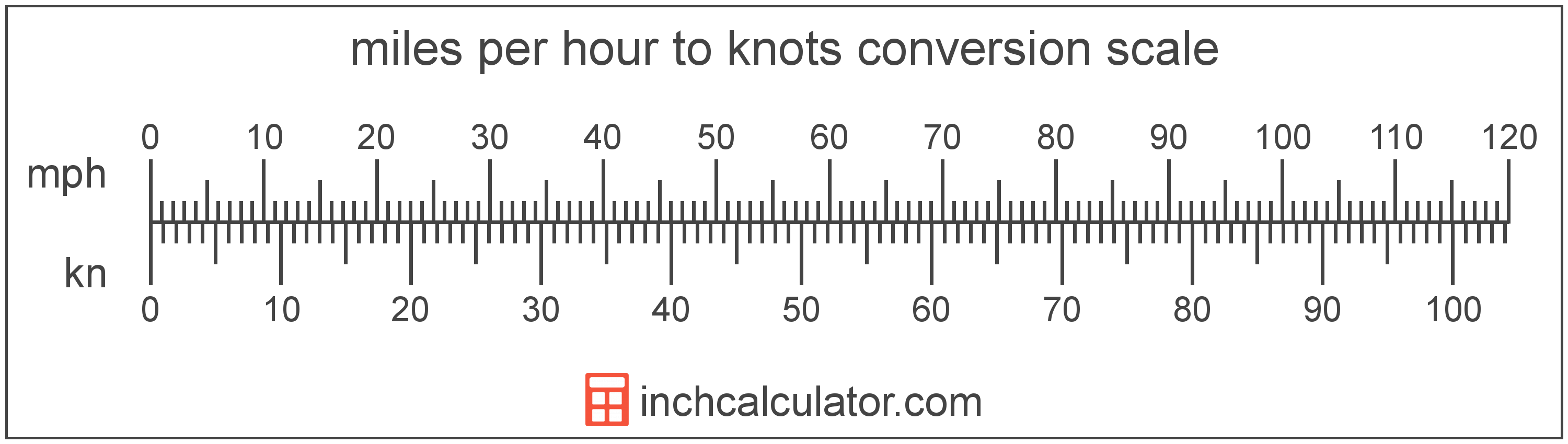# Miles per Hour to Knots Converter

Enter the speed in miles per hour below to get the value converted to knots.

Results in Knots:1 mph = 0.868976 kn

Do you want to convert knots to miles per hour?

## How to Convert Miles per Hour to Knots

To convert a measurement in miles per hour to a measurement in knots, multiply the speed by the following conversion ratio: 0.868976 knots/mile per hour.

Since one mile per hour is equal to 0.868976 knots, you can use this simple formula to convert:

knots = miles per hour × 0.868976

The speed in knots is equal to the speed in miles per hour multiplied by 0.868976.

For example, here's how to convert 5 miles per hour to knots using the formula above.
knots = (5 mph × 0.868976) = 4.344881 knMiles per hour and knots are both units used to measure speed. Calculate speed in miles per hour or knots using our speed calculator or keep reading to learn more about each unit of measure.

## What Are Miles per Hour?

Miles per hour are a measurement of speed expressing the distance traveled in miles in one hour.

The mile per hour is a US customary and imperial unit of speed. Miles per hour can be abbreviated as mph, and are also sometimes abbreviated as mi/h or MPH. For example, 1 mile per hour can be written as 1 mph, 1 mi/h, or 1 MPH.

Miles per hour can be expressed using the formula:
vmph = dmi / thr

The velocity in miles per hour is equal to the distance in miles divided by time in hours.

## What is a Knot?

One knot is equal to a speed of one nautical mile per hour, or one minute of latitude per hour.

Knots can be abbreviated as kn, and are also sometimes abbreviated as kt. For example, 1 knot can be written as 1 kn or 1 kt.

## Mile per Hour to Knot Conversion Table

Table showing various mile per hour measurements converted to knots.
Miles Per Hour Knots
1 mph 0.868976 kn
2 mph 1.738 kn
3 mph 2.6069 kn
4 mph 3.4759 kn
5 mph 4.3449 kn
6 mph 5.2139 kn
7 mph 6.0828 kn
8 mph 6.9518 kn
9 mph 7.8208 kn
10 mph 8.6898 kn
11 mph 9.5587 kn
12 mph 10.43 kn
13 mph 11.3 kn
14 mph 12.17 kn
15 mph 13.03 kn
16 mph 13.9 kn
17 mph 14.77 kn
18 mph 15.64 kn
19 mph 16.51 kn
20 mph 17.38 kn
21 mph 18.25 kn
22 mph 19.12 kn
23 mph 19.99 kn
24 mph 20.86 kn
25 mph 21.72 kn
26 mph 22.59 kn
27 mph 23.46 kn
28 mph 24.33 kn
29 mph 25.2 kn
30 mph 26.07 kn
31 mph 26.94 kn
32 mph 27.81 kn
33 mph 28.68 kn
34 mph 29.55 kn
35 mph 30.41 kn
36 mph 31.28 kn
37 mph 32.15 kn
38 mph 33.02 kn
39 mph 33.89 kn
40 mph 34.76 kn

## References

1. Wikipedia, Miles per hour, https://en.wikipedia.org/wiki/Miles_per_hour
2. NASA, Knots Versus Miles per Hour, https://www.grc.nasa.gov/WWW/K-12/WindTunnel/Activities/knots_vs_mph.html